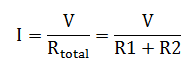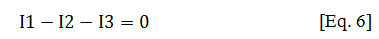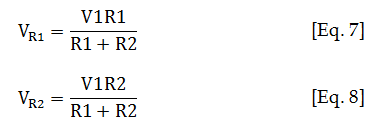# Circuit Analysis Methods

There are several circuit analysis methods. In this article I will discuss the most common. In the proceeding discussion I will just use a resistor as an example but in actual application it is not limited to it.

## Popular Circuit Analysis Methods

### 1. Ohm’s Law

Ohm’s law is very popular in resistive circuit. It states that the current flowing through a conductor with a finite resistance is directly proportional to the applied voltage and inversely proportional to the resistance. Hence, for a circuit with an applied voltage V and a resistance R, the current will beIf the circuit is complex, the resistance should be the total or the equivalent circuit resistance. Supposing there are two resistances R1 and R2 connected in series and a voltage supply V, the current of the circuit will be### 2. Kirchhoff’s Voltage Law (KVL)

Another very useful tool in analyzing electrical and electronic circuitry  is the Kirchhoff’s voltage law. According to this law, the sum of the voltage in a close loop is equal to zero. To illustrate this well, consider the circuit in Figure 9. The sum of the input voltage V, the voltage drop across R1 and voltage of R2 is zero. To do this, we will make a close loop which starts from the input V going to R1 then to R2 and the circuit ground. Input voltage V is the circuit source and both R1 and R2 are the loads, so the current direction is going right as indicated in Figure 9. We will start the loop as follow;Re-arranging the equation and expressing it in terms of current,If you notice, this is the same with Ohm’s law previously.Figure 9 – Circuit model for KVL analysis. In KVL, the sum of the voltages in a close loop is equal to zero. In layman’s explanation on the above circuit, the sum of all the voltage drops (R1 and R2) is always equal to the value of the input voltage (V).

### 3. Kirchhoff’s Current Law

Kirchhoff’s current law or KCL states that the sum of the current leaving and entering a node is equal to zero. A node is a point in a circuit wherein currents are leaving and entering. In Figure 10, point A is a node.

By following KCL, the sum of the currents at node A is equal to zero. Let’s assume the current entering as positive while the current leaving is negative (you can assume otherwise though). So,Figure 10 – Circuit model for KCL analysis. In this method, the sum of the current in a node is zero.

### 4. Voltage Division Theorem

The individual voltage drop of resistors connected in series is can be derived using KVL or mesh method. To refresh, KVL stands for Kirchhoff’s Voltage Law which states that the algebraic sum of the voltage in a single loop is equal to zero. Although KVL is an effective way in doing the task but it may consume longer time, but with the adoption of voltage divider theorem or simply VDT the individual voltage is very easy to determine and needs only short period of time.

Voltage divider theorem is applicable to series circuit. The voltage drop of a resistor R is equal to the product of the circuit supply voltage and the resistance R divided by the total circuit resistance. Since this is a series circuit, the total resistance is just the sum of the resistances. Considering the circuit in Figure 11, the voltage drop of R1 and R2 by using VDT isFigure 11 – Circuit model for voltage division theorem. VDT is a short cut method for determining the voltage drop of a particular resistor in a series connection.

### 5. Current Division Theorem

In VDT, the circuit in reference is a series.The individual voltage drop is equal to the product of the circuit supply voltage and the resistance of the subject resistor (the resistor in which you want to get the voltage drop) divided by the total resistance. Meanwhile, current division theorem or CDT is applicable to a parallel circuit. So in Figure 12, the current of R1 and R2 isThis site uses Akismet to reduce spam. Learn how your comment data is processed.